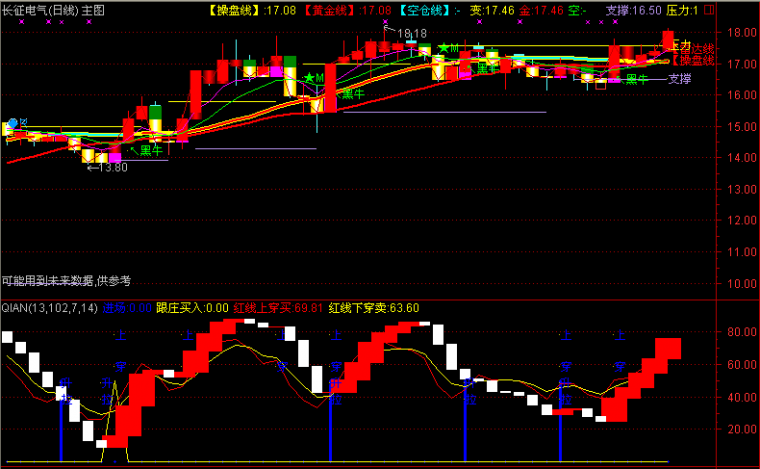﻿ 无敌抄底选股-通达信公式 －程序化交易（CXH99.COM）

# 无敌抄底选股[通达信公式]

A1:=(C-LLV(L,9))/(HHV(H,9)-LLV(L,9))*100;
A2:=SMA(A1,3,1);
A3:=SMA(A2,3,1);
A4:=3*A2-2*A3;
X:=LLV(A4,2)=LLV(A4,8);
MA13:=MA(C,13),COLORWHITE;
MA34:=MA(C,34),COLORYELLOW;
MA55:=MA(C,55),COLORRED;
YY1:=O<MA13 AND C>MA13;
YY2:=O<MA34 AND C>MA34;
YY3:=O<MA55 AND C>MA55;
YY4:=C/O>=1.04;
YY5:=V>HHV(H,10);
YY6:=MA13>=REF(MA13,1);(注：由于人数限制，QQ或微信请选择方便的一个联系我们就行，加好友时请简单备注下您的需求，否则无法通过。谢谢您！)

【字体： 】【打印文章】【查看评论

没有相关内容